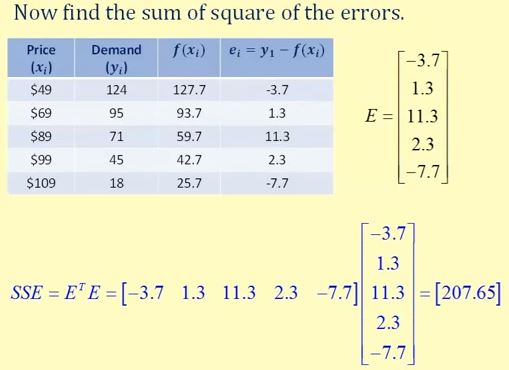### Introduction

For points (x1, y1), (x2, y2), ... (xn, yn), the least square regression line can be given by:

f(x) = b + mx

which will minimize the sum of the squared error, which are the error in using the regression function f(x) to estimate the true y values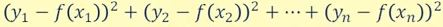where ei = yi - f(xi) is the error approximating yi .

Let's see if we can set this up as a system of equations and then solve using matrices.

Using our points (x1, y1), (x2, y2), ... (xn, yn) we would have the following system of equations: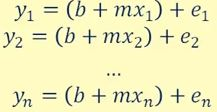Now let's set up an matrix equation. Let: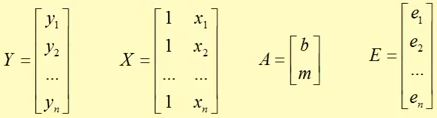This gives us the matrix equation: Y = XA + E.

We now just need to solve this for A.

The solution to least square regression equation Y = XA + E is: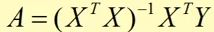The sum of the squared errors (SSE) is: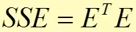### Example

Example: Determine the least squares regression line using a matrics. The price is \$x and y is the monthly sales. Then find the sum of the squared errors.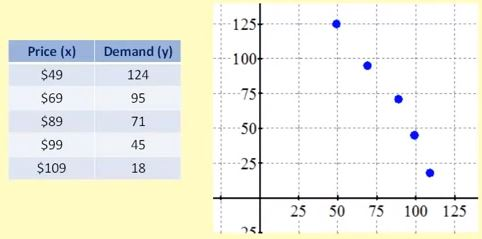The solution is to work out A using:Step 1: Get the matrices Y and X.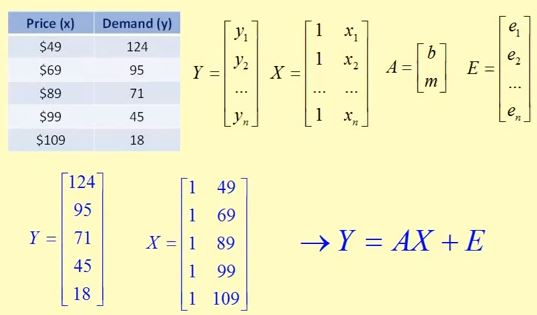Step 2: Work out the XTX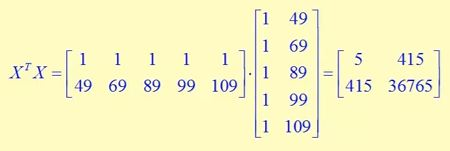Step 3: Find the inverse of XTX. (The tutorial about the Inverse is here)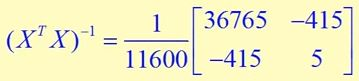Step 4: Find the XTY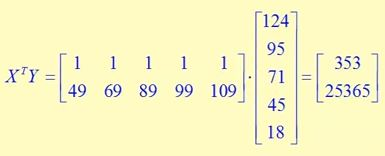Step 5: Finally, the result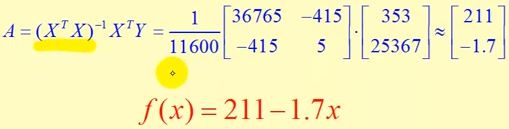and the Squared Error is